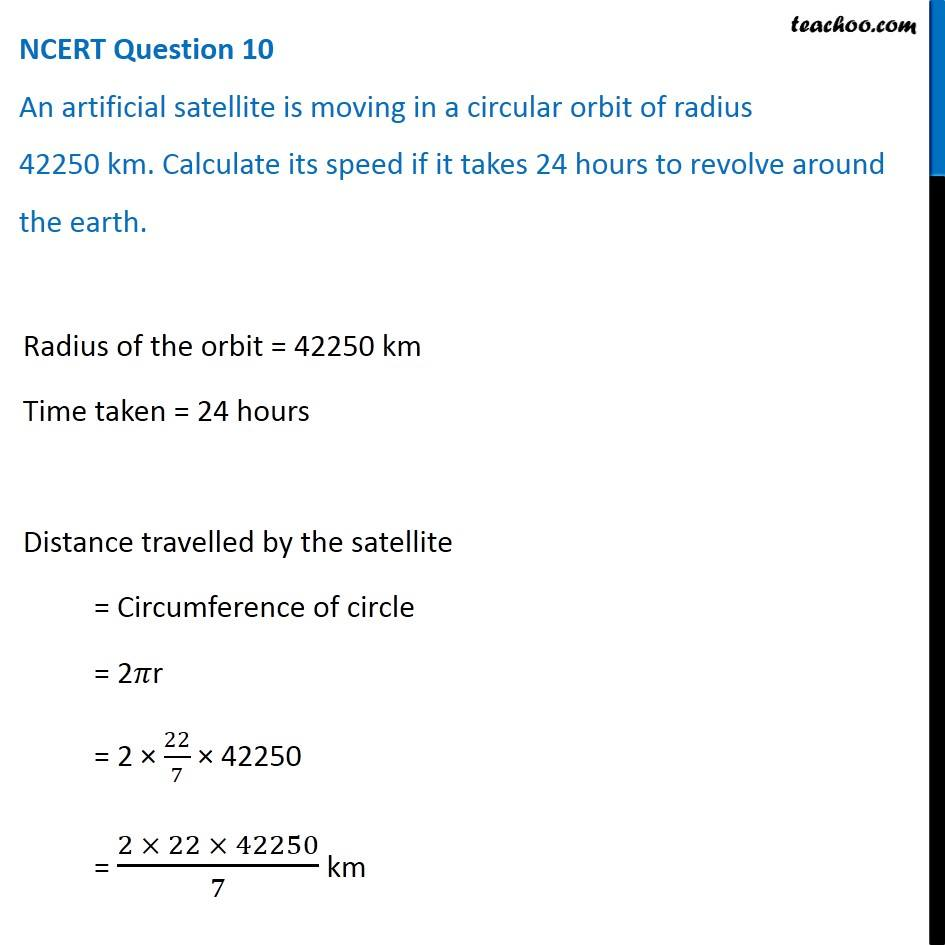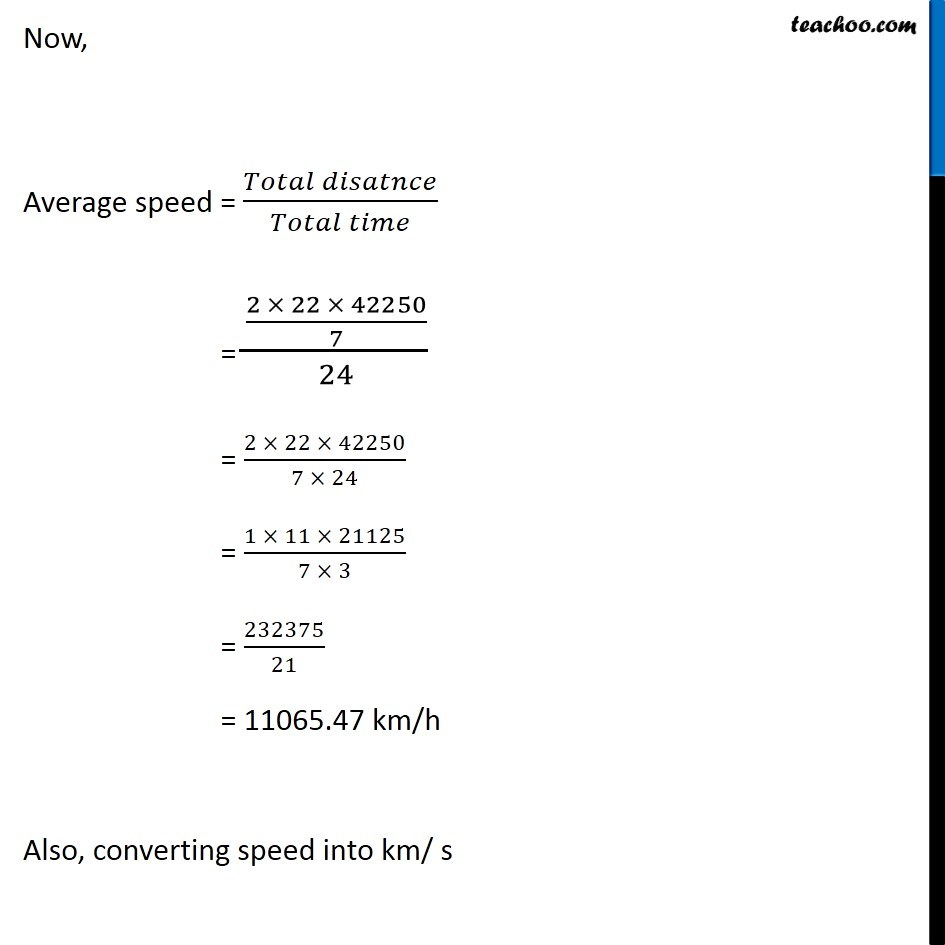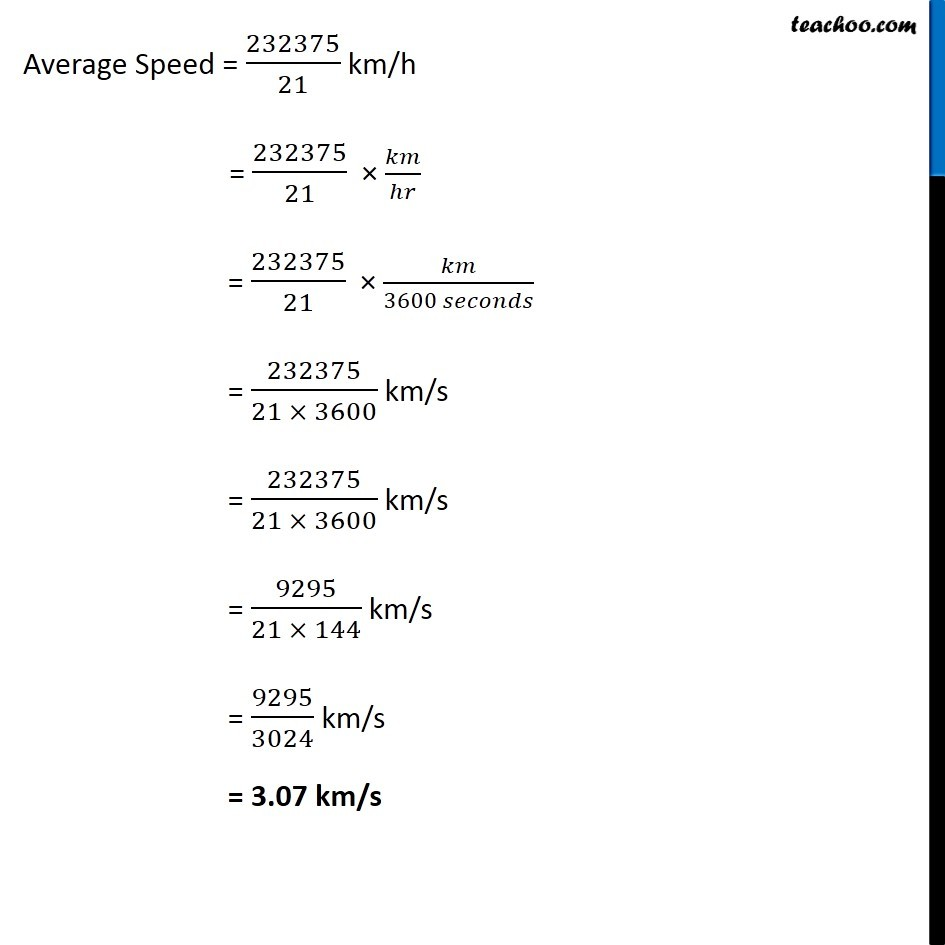NCERT Questions

Class 9
Chapter 8 Class 9 - MotionGet live Maths 1-on-1 Classs - Class 6 to 12

### Transcript

NCERT Question 10 An artificial satellite is moving in a circular orbit of radius 42250 km. Calculate its speed if it takes 24 hours to revolve around the earth. Radius of the orbit = 42250 km Time taken = 24 hours Distance travelled by the satellite = Circumference of circle = 2𝜋r = 2 × 22/7 × 42250 = (2 × 22 × 42250)/7 km Radius of the orbit = 42250 km Time taken = 24 hours Distance travelled by the satellite = Circumference of circle = 2𝜋r = 2 × 22/7 × 42250 = (2 × 22 × 42250)/7 km Average Speed = 232375/21 km/h = 232375/21 × 𝑘𝑚/ℎ𝑟 = 232375/21 × 𝑘𝑚/(3600 𝑠𝑒𝑐𝑜𝑛𝑑𝑠) = 232375/(21 × 3600) km/s = 232375/(21 × 3600) km/s = 9295/(21 × 144) km/s = 9295/3024 km/s = 3.07 km/s